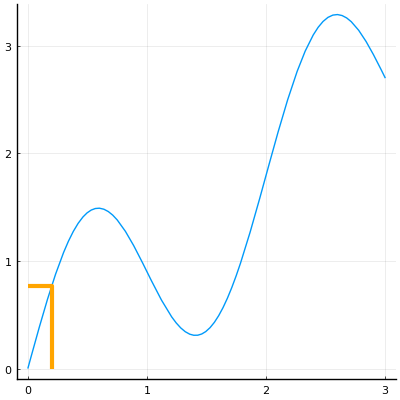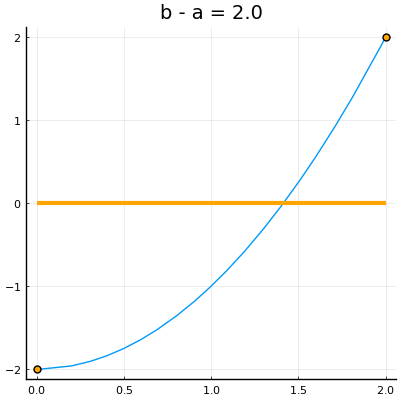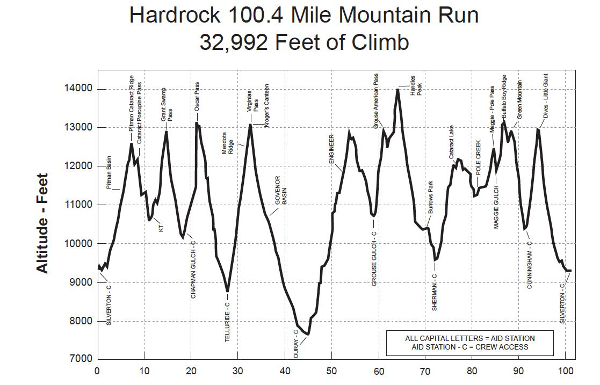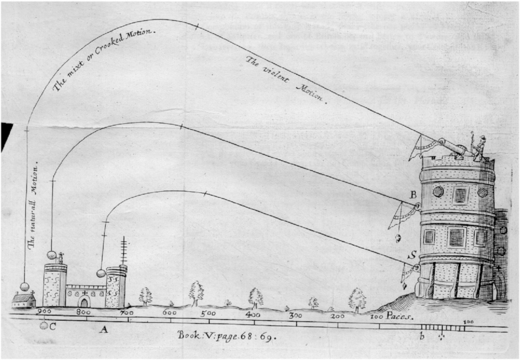# Implications of continuity

Continuity for functions is a valued property which carries implications. In this section we discuss two: the intermediate value theorem and the extreme value theorem. These two theorems speak to some fundamental applications of calculus: finding zeros of a function and finding extrema of a function.

## Intermediate Value Theorem

The intermediate value theorem: If $f$ is continuous on $[a,b]$ with, say, $f(a) < f(b)$, then for any $y$ with $f(a) < y < f(b)$ there exists a $c$ in $[a,b]$ with $f(c) = y$.Illustration of intermediate value theorem. The theorem implies that any randomly chosen $y$ value between $f(a)$ and $f(b)$ will have at least one $x$ in $[a,b]$ with $f(x)=y$.

In the early years of calculus, the intermediate value theorem was intricately connected with the definition of continuity, now it is a consequence.

The basic proof starts with a set of points in $[a,b]$: $C = \{x \text{ in } [a,b] \text{ with } f(x) \leq y\}$. The set is not empty (as $a$ is in $C$) so it must have a largest value, call it $c$ (this requires the completeness property of the real numbers). By continuity of $f$, it can be shown that $\lim_{x \rightarrow c-} f(x) = f(c) \leq y$ and $\lim_{y \rightarrow c+}f(x) =f(c) \geq y$, which forces $f(c) = y$.

### Bolzano and the bisection method

Suppose we have a continuous function $f(x)$ on $[a,b]$ with $f(a) < 0$ and $f(b) > 0$. Then as $f(a) < 0 < f(b)$, the intermediate value theorem guarantees the existence of a $c$ in $[a,b]$ with $f(c) = 0$. This was a special case of the intermediate value theorem proved by Bolzano first. Such $c$ are called zeros of the function $f$.

We use this fact when a building a "sign chart" of a continous function. Between any two consecutive zeros the function can not change sign. (Why?) So a "test point" can be used to determine the sign of the function over an entire interval.

Here, we use the Bolzano theorem to give an algorithm - the bisection method - to locate the value $c$ under the assumption $f$ is continous on $[a,b]$ and changes sign between $a$ and $b$.Illustration of the bisection method to find a zero of a function. At each step the interval has $f(a)$ and $f(b)$ having opposite signs so that the intermediate value theorem guaratees a zero.

Call $[a,b]$ a bracketing interval if $f(a)$ and $f(b)$ have different signs. We remark that having different signs can be expressed mathematically as $f(a) \cdot f(b) < 0$.

We can narrow down where a zero is in $[a,b]$ by following this recipe:

• Pick a midpoint of the interval, for concreteness $c = (a+b)/2$.

• If $f(c) = 0$ we are done, having found a zero in $[a,b]$.

• Otherwise if must be that either $f(a)\cdot f(c) < 0$ or $f(c) \cdot f(b) < 0$. If $f(a) \cdot f(c) < 0$, then let $b=c$ and repeat the above. Otherwise, let $a=c$ and repeat the above.

At each step the bracketing interval is narrowed, indeed split in half as defined, or a zero is found.

For the real numbers this algorithm never stops unless a zero is found. A "limiting" process is used to say that if it doesn't stop, it will converge to some value.

However, using floating point numbers leads to differences from the real-number situation. In this case, due to the ultimate granularity of the approximation of floating point values to the real numbers, the bracketing interval eventually can't be subdivided, that is no $c$ is found over the floating point numbers with $a < c < b$. So there is a natural stopping criteria: stop when there is an exact zero, or when the bracketing interval gets too small.

We can write a relatively simple program to implement this algorithm:

function bisection(f, a, b)
if f(a) == 0 return(a) end
if f(b) == 0 return(b) end
if f(a) * f(b) > 0 error("[a,b] is not a bracketing interval") end

tol = 1e-14  # small number (but should depend on size of a, b)
c = a/2 + b/2

while abs(b-a) > tol
if f(c) == 0 return(c) end

if f(a) * f(c) < 0
a, b = a, c
else
a, b = c, b
end

c = a/2 + b/2

end
c
end

bisection (generic function with 1 method)


This function uses a while loop to repeat the process of subdividing $[a,b]$. A while loop will repeat until the condition is no longer true. The above will stop for reasonably sized floating point values (within $(-100, 100)$, say). The value $c$ returned need not be an exact zero. Let's see:

c = bisection(sin, 3, 4)

3.141592653589793


This value of $c$ is a floating-point approximation to $\pi$, but is not quite a zero:

sin(c)

1.2246467991473532e-16


(Even pi itself is not a "zero" due to floating point issues.)

### The find_zero function.

The Roots package has a function find_zero that implements the bisection method when called as find_zero(f, (a, b)) where $[a,b]$ is a bracket. Its use is similar to bisection above. This package is loaded when CalculusWithJulia is. We illlustrate the usage of find_zero in the following:

using CalculusWithJulia  # loads Roots
using Plots
find_zero(sin, (3, 4))   # use a tuple, (a, b), to specify the bracketing interval

3.1415926535897936


This function utilizes some facts about floating point values to guarantee that the answer will be a zero or the product of the function value at the floating point values just to the left and right will be negative. No specification of a tolerance is needed.

##### Example

The polynomial $f(x) = x^5 - x + 1$ has a zero between $-2$ and $-1$. Find it.

f(x) = x^5 - x + 1
c = find_zero(f, (-2, -1))
(c, f(c))

(-1.1673039782614185, 1.3322676295501878e-15)


We see, as before, that $f(c)$ is not quite $0$. (But you can check that f(prevfloat(c)) is negative, while f(c) is seen to be positive.)

##### Example

The function $f(x) = e^x - x^4$ has a zero between $5$ and $10$, as this graph shows:

f(x) = exp(x) - x^4
plot(f, 5, 10)


Find the zero numerically. The plot shows $f(5) < 0 < f(10)$, so $[5,10]$ is a bracket. We thus have:

find_zero(f, (5, 10))

8.6131694564414

##### Example

Find all real zeros of $f(x) = x^3 -x + 1$ using the bisection method.

A plot will show us a bracketing interval:

f(x) = x^3 - x + 1
plot(f, -3, 3)


It appears (and a plot over $[0,1]$ verifies) that there is one zero between $-2$ and $-1$. It is found with:

find_zero(f, (-2, -1))

-1.3247179572447458

##### Example

The equation $\cos(x) = x$ has just one solution, as can be seen in this plot:

f(x) = cos(x)
g(x) = x
plot(f, -pi, pi)
plot!(g)


Find it.

We see from the graph that it is clearly between $0$ and $2$, so all we need is a function. (We have two.) The trick is to observe that solving $f(x) = g(x)$ is the same problem as solving for $x$ where $f(x) - g(x) = 0$. So we define the difference and use that:

h(x) = f(x) - g(x)
find_zero(h, (0, 2))

0.7390851332151607

##### Example

We wish to compare two trash collection plans

• Plan 1: You pay 47.49 plus 0.77 per bag.

• Plan 2: You pay 30.00 plus 2.00 per bag.

There are some cases where plan 1 is cheaper and some where plan 2 is. Categorize them.

Both plans are linear models and may be written in slope-intercept form:

plan1(x) = 47.49 + 0.77x
plan2(x) = 30.00 + 2.00x

plan2 (generic function with 1 method)


Assuming this is a realistic problem and an average American household might produce 10-20 bags of trash a month (yes, that seems too much!) we plot in that range:

plot(plan1, 10, 20)
plot!(plan2)


We can see the intersection point is around 14 and that if a family generates between 0-14 bags of trash per month that plan 2 would be cheaper.

Let's get a numeric value, using a simple bracket and an anonymous function:

find_zero(x -> plan1(x) - plan2(x), (10, 20))

14.21951219512195

##### Example, the flight of an arrow

The flight of an arrow can be modeled using various functions, depending on assumptions. Suppose an arrow is launched in the air from a height of 0 feet above the ground at an angle of $\theta = \pi/4$. With a suitable choice for the initial velocity, a model without wind resistance for the height of the arrow at a distance $x$ units away may be:

$~ j(x) = \tan(\theta) x - (1//2) \cdot g(\frac{x}{v_0 \cos\theta})^2. ~$

In julia we have, taking $v_0=200$:

j(x; theta=pi/4, g=32, v0=200) = tan(theta)*x - (1/2)*g*(x/(v0*cos(theta)))^2

j (generic function with 1 method)


With a velocity-dependent wind resistance given by $\gamma$, again with some units, a similar equation can be constructed. It takes a different form:

$~ y(x) = (\frac{g}{\gamma v_0 \cos(\theta)} + \tan(\theta)) \cdot x + \frac{g}{\gamma^2}\log(\frac{v_0\cos(\theta) - \gamma x}{v_0\cos(\theta)}) ~$

Again, $v_0$ is the initial velocity and is taken to be $200$ and $\gamma$ a resistance, which we take to be $1$. With this, we have the following julia definition (with a slight reworking of $\gamma$):

function y(x; theta=pi/4, g=32, v0=200, gamma=1)
a = gamma * v0 * cos(theta)
(g/a + tan(theta)) * x + g/gamma^2 * log((a-gamma^2 * x)/a)
end

y (generic function with 1 method)


For each model, we wish to find the value of $x$ after launching where the height is modeled to be 0. That is how far will the arrow travel before touching the ground?

For the model without wind resistance, we can graph the function easily enough. Let's guess the distance is no more than 500 feet:

plot(j, 0, 500)


Well, we haven't even seen the peak yet. Better to do a little spade work first. This is a quadratic function, so we can use roots from SymPy to find the roots:

@vars x
roots(j(x))

Dict{Any,Any} with 2 entries:
0                => 1
1250.00000000000 => 1


We see that $1250$ is the largest root. So we plot over this domain to visualize the flight:

plot(j, 0, 1250)


As for the model with wind resistance, a quick plot over the same interval, $[0, 1250]$ yields:

plot(y, 0, 1250)


Oh, "Domain Error." Of course, when the argument to the logarithm is negative we will have issues. We don't have the simplicity of using poly_roots to find out the answer, so we solve for when $a-\gamma^2 x$ is $0$:

gamma = 1
a = 200 * cos(pi/4)
b = a/gamma^2

141.4213562373095


We try on the reduced interval avoiding the obvious asymptote at b by subtracting $1$:

plot(y, 0, b - 1)


Now we can see the zero is around 140. A simple bracket will be $[b/2, b-1]$, so we cam solve:

x1 = find_zero(y, (b/2, b-1/10))

140.7792933802306


The answer is approximately $140.7$

Finally, we plot both graphs at once to see that it was a very windy day indeed.

plot(j, 0, 1250)
plot!(y, 0, x1)

##### Example: bisection and non-continuity

The Bolzano theorem assumes a continuous function $f$, and when applicable, yields an algorithm to find a guaranteed zero. However, the algorithm itself does not know that the function is continuous or not, only that the function changes sign. As such, it can produce answers that are not "zeros" when used with discontinuous functions. However, this can still be fruitful, as the algorithm will yield information about crossing values of $0$, possibly at discontinuities.

For example, let $f(x) = 1/x$. Clearly the interval $[-1,1]$ is a "bracketing" interval as $f(x)$ changes sign between $a$ and $b$. What does the algorithm yield:

f(x) = 1/x
x0 = find_zero(f, (-1, 1))

0.0


The function is not defined at the answer, but we do have the fact that just to the left of the answer (prevfloat) and just to the right of the answer (nextfloat) the function changes sign:

sign(f(prevfloat(x0))), sign(f(nextfloat(x0)))

(-1.0, 1.0)


So, the "bisection method" applied here finds a point where the function crosses $0$, either by continuity or by jumping over the $0$. (A jump discontinuity at $x=c$ is defined by the left and right limits of $f$ at $c$ existing but being unequal. The algorithm can find $c$ when this type of function jumps over $0$.)

### The find_zeros function

The bisection method suggests a naive means to search for all zeros within an interval $(a, b)$: split the interval into many small intervals and for each that is a bracketing interval find a zero. This simple description has three flaws: it might miss values where the function doesn't't actually cross the $x$ axis; it might miss values where the function just dips to the other side; and it might miss multiple values in the same small interval.

Still, with some engineering, this can be a useful approach, save the caveats. This idea is implemented in the find_zeros function of the Roots package. The function is called via find_zeros(f, a, b) but here the interval $[a,b]$ is not necessarily a bracketing interval.

To see, we have:

f(x) = cos(10*pi*x)
find_zeros(f, 0, 1)

10-element Array{Float64,1}:
0.05
0.15
0.25
0.35
0.45
0.5499999999999999
0.6499999999999999
0.75
0.85
0.95


Or for a polynomial:

f(x) = x^5 - x^4 + x^3 - x^2 + 1
find_zeros(f, -10, 10)

1-element Array{Float64,1}:
-0.6518234538234416


(Here $-10$ and $10$ were arbitrarily chosen. Cauchy's method could be used to be more systematic.)

##### Solving f(x) = g(x)

Use find_zeros to find when $e^x = x^5$ in the interval $[-20, 20]$. Verify the answers.

To proceed with find_zeros, we define $f(x) = e^x - x^5$, as $f(x) = 0$ precisely when $e^x = x^5$. The zeros are then found with:

f(x) = exp(x) - x^5
zs = find_zeros(f, -20, 20)

2-element Array{Float64,1}:
1.2958555090953687
12.713206788867632


The output of find_zeros is a vector of values. To check that each value is an approximate zero can be done with the "." (broadcast) syntax:

f.(zs)

2-element Array{Float64,1}:
0.0
0.0


(For a continuous function this should be the case that the values returned by find_zeros are approximate zeros. Bear in mind that if $f$ is not continous the algorithm might find jumping points that are not zeros and may not even be in the domain of the function.)

## Extreme value theorem

The Extreme Value Theorem is another consequence of continuity.

To discuss the extreme value theorem, we define an absolute maximum of $f(x)$ over an interval $I$ to be a value $f(c)$, $c$ in $I$, where $f(x) \leq f(c)$ for any $x$ in $I$. Similarly, an absolute minimum of $f(x)$ over an interval $I$ can be defined.

This chart of the Hardrock 100 illustrates the two concepts.Illustration of the bisection method to find a zero of a function. At each step the interval has $f(a)$ and $f(b)$ having opposite signs so that the intermediate value theorem guaratees a zero.

The extreme value theorem discusses an assumption that ensures such absolute maximum and absolute minimum values exist.

The extreme value theorem: If $f(x)$ is continuous over a closed interval $[a,b]$ then $f$ has an absolute maximum and an absolute minimum over $[a,b]$.

(By continuous over $[a,b]$ we mean continuous on $(a,b)$ and right continuous at $a$ and left continuous at $b$.)

The assumption that $[a,b]$ includes its endpoints (it is closed) is crucial to make a guarantee. There are functions which are continuous on open intervals for which this result is not true. For example, $f(x) = 1/x$ on $(0,1)$. This function will have no smallest value or largest value, as defined above.

The extreme value theorem is an important theoretical tool for investigating maxima and minima of functions.

##### Example

The function $f(x) = \sqrt{1-x^2}$ is continuous on the interval $[-1,1]$ (in the sense above). It then has an absolute maximum, we can see to be $1$ occurring at an interior point $0$. The absolute minimum is $0$, it occurs at each endpoint.

##### Example

The function $f(x) = x \cdot e^{-x}$ on the closed interval $[0, 5]$ is continuous. Hence it has an absolute maximum, which a graph shows to be $0.4$. It has an absolute minimum, clearly the value $0$ occurring at the endpoint.

f(x) = x * exp(-x)
plot(f, 0, 5)

##### Example

The tangent function does not have a guarantee of absolute maximum or minimum over $(-\pi/2, \pi/2)$, as it is not continuous at the endpoints. In fact, it doesn't have either extrema - it has vertical asymptotes at each.

##### Example

The function $f(x) = x^{2/3}$ over the interval $[-2,2]$ has cusp at $0$. However, it is continuous on this closed interval, so must have an absolute maximum and absolute minimum. They can be seen from the graph to occur at the endpoints and the cusp at $x=0$, respectively:

f(x) = (x^2)^(1/3)
plot(f, -2, 2)


(The definition x^(2/3) fails, can you see why?)

##### Example

A New York Times article discusses an idea of Norway moving its border some 490 feet north and 650 feet east in order to have the peak of Mount Halti be the highest point in Finland, as currently it would be on the boundary. Mathematically this hints at a higher dimensional version of the extreme value theorem.

## Questions

###### Question

There is negative zero in the interval $[-10, 0]$ for the function $f(x) = e^x - x^4$. Find its value numerically:

###### Question

There is zero in the interval $[0, 5]$ for the function $f(x) = e^x - x^4$. Find its value numerically:

###### Question

Let $f(x) = x^2 - 10 \cdot x \cdot \log(x)$. This function has two zeros on the positive $x$ axis. You are asked to find the largest (graph and bracket...).

###### Question

The airyai function has infinitely many negative roots, as the function oscillates when $x < 0$ and no positive roots. Find the second largest root using the graph to bracket the answer, and then solve.

plot(airyai, -10, 10)   # airyai loaded in SpecialFunctions by CalculusWithJulia


The second largest root is:

###### Question

(From Strang, p. 37)

Certainly $x^3$ equals $3^x$ at $x=3$. Find the largest value for which $x^3 = 3x$.

Compare $x^2$ and $2^x$. They meet at $2$, where do the meet again?

Just by graphing, find a number in $b$ with $2 < b < 3$ where for values less than $b$ there is a zero beyond $b$ of $b^x - x^b$ and for values more than $b$ there isn't.

###### Question <small>What goes up must come down...</small>Illustration of the bisection method to find a zero of a function. At each step the interval has $f(a)$ and $f(b)$ having opposite signs so that the intermediate value theorem guaratees a zero.

In 1638, according to Amir D. Aczel, an experiment was performed in the French Countryside. A monk, Marin Mersenne, launched a cannonball straight up into the air in an attempt to help Descartes prove facts about the rotation of the earth. Though the experiment was not successful, Mersenne later observed that the time for the cannonball to go up was greater than the time to come down. "Vertical Projection in a Resisting Medium: Reflections on Observations of Mersenne".

This isn't the case for simple ballistic motion where the time to go up is equal to the time to come down. We can "prove" this numerically. For simple ballistic motion:

$~ f(t) = -(1/2)\cdot 32 t^2 + v_0t. ~$

The time to go up and down are found by the two zeros of this function. The peak time is related to a zero of a function given by D(f), which for now we'll take as a mystery function, but later will be known as the derivative. Here is its definition:

using ForwardDiff
D(f) = x -> ForwardDiff.derivative(f, x)


Let $v_0= 390$. The three times in question can be found from the zeros of f and D(f). What are they?

###### Question <small>What goes up must come down... (again)</small>

For simple ballistic motion you find that the time to go up is the time to come down. For motion within a resistant medium, such as air, this isn't the case. Suppose a model for the height as a function of time is given by

$~ h(t) = (\frac{g}{\gamma^2} + \frac{v_0}{\gamma})(1 - e^{-\gamma t}) - \frac{gt}{\gamma} ~$

Here $g=32$, again we take $v_0=390$, and $\gamma$ is a drag coefficient that we will take to be $1$. This is valid when $h(t) \geq 0$. In Julia, rather than hard-code the parameter values, for added flexibility we can pass them in as keyword arguments:

h(t; g=32, v0=390, gamma=1) = (g/gamma^2 + v0/gamma)*(1 - exp(-gamma*t)) - g*t/gamma

h (generic function with 1 method)


Now find the three times: $t_0$, the starting time; $t_a$, the time at the apex of the flight; and $t_f$, the time the object returns to the ground.

###### Question

Part of the proof of the intermediate value theorem rests on knowing what the limit is of $f(x)$ when $f(x) > y$ for all $x$. What can we say about $L$ supposing $L = \lim_{x \rightarrow c+}f(x)$ under this assumption on $f$?

###### Question

The extreme value theorem has two assumptions: a continuous function and a closed interval. Which of the following examples fails to satisfy the consequence of the extreme value theorem because the interval is not closed? (The consequence - the existence of an absolute maximum and minimum - can happen even if the theorem does not apply.)

###### Question

The extreme value theorem has two assumptions: a continuous function and a closed interval. Which of the following examples fails to satisfy the consequence of the extreme value theorem because the function is not continuous?

###### Question

The extreme value theorem has two assumptions: a continuous function and a closed interval. Which of the following examples fails to satisfy the consequence of the extreme value theorem because the function is not continuous?

###### Question

The function $f(x) = x^3 - x$ is continuous over the interval $I=[-2,2]$. Find a value $c$ for which $M=f(c)$ is an absolute maximum over $I$.

###### Question

The function $f(x) = x^3 - x$ is continuous over the interval $I=[-1,1]$. Find a value $c$ for which $M=f(c)$ is an absolute maximum over $I$.

###### Question

Consider the continuous function $f(x) = \sin(x)$ over the closed interval $I=[0, 10\pi]$. Which of these is true?

###### Question

Consider the continuous function $f(x) = \sin(x)$ over the closed interval $I=[0, 10\pi]$. Which of these is true?

###### Question

The extreme value theorem says that on a closed interval a continuous function has an extreme value $M=f(c)$ for some $c$. Does it also say that $c$ is unique? Which of these examples might help you answer this?

##### Question

The zeros of the equation $\cos(x) \cdot \cosh(x) = 1$ are related to vibrations of rods. Using find_zeros, what is the largest zero in the interval $[0, 6\pi]$?

##### Question

A parametric equation is specified by a parameterization $(f(t), g(t)), a \leq t \leq b$. The parameterization will be continuous if and only if each function is continuous.

Suppose $k_x$ and $k_y$ are positive integers and $a, b$ are positive numbers, will the Lissajous curve given by $(a\cos(k_x t), b\sin(k_y t))$ be continuous?

Here is a sample graph for $a=1, b=2, k_x=3, k_y=4$:

a,b = 1, 2
k_x, k_y = 3, 4
plot(t -> a * cos(k_x *t), t-> b * sin(k_y * t), 0, 4pi)